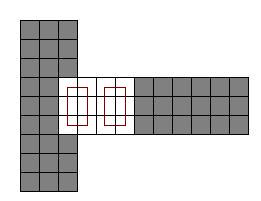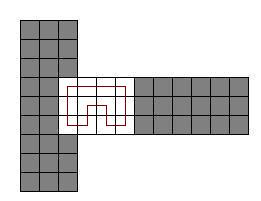### Problem Statement

You are given a 3x3x3 cube. Each face of the cube contains a 3x3 grid of nine squares. Some of the squares are painted and the rest are unpainted. Your task is to calculate the number of ways you can cover all the unpainted squares with "cube-cycles".

A cube-cycle is a sequence of 3 or more squares, where each square is "cube-adjacent" to the next square, and the last square is "cube-adjacent" to the first square. All squares in a cube-cycle must be distinct. Two squares are cube-adjacent if they share a common edge. That common edge can be on a face or on an edge of the cube. Each square in the cube has exactly four cube-adjacent squares.

Note that two cube-cycles might be distinct even if they contain the same set of squares. See example 0.

You are given the cube as a String[] containing 54 characters, where each character represents a single square. The String[] will be formatted as a net of the cube, as shown below:

```+---+
|   |
+---+---+---+---+
|   |   |   |   |
+---+---+---+---+
|   |
+---+```

Painted squares are represented by '*' characters and unpainted squares are represented by '.' characters. You are only allowed to cover unpainted squares with cube-cycles. You are not allowed to cover painted squares. All unpainted squares must be covered, and no two cube-cycles can overlap each other. Return the number of ways you can cover the cube modulo 1,000,000,007.

### Definition

 Class: CubePainting Method: count Parameters: String[] Returns: int Method signature: int count(String[] cube) (be sure your method is public)

### Constraints

-

cube will contain exactly 9 elements.

-

Elements 0, 1, 2, 6, 7 and 8 of cube will each contain exactly 3 characters.

-

Elements 3, 4 and 5 of cube will each contain exactly 12 characters.

-

All characters in cube will be either '*' or '.'.

### Examples

0)

 ```{"***", "***", "***", "**....******", "**....******", "**....******", "***", "***", "***"}```
`Returns: 3`
 There are two ways to cover it with one cycle and one way with two cycles.1)

 ```{"***", "***", "***", "************", "............", "************", "***", "***", "***"}```
`Returns: 1`
 There is only one way to cover a strip.
2)

 ```{".*.", "***", ".*.", ".*..*..*..*.", "************", ".*..*..*..*.", ".*.", "***", ".*."}```
`Returns: 1`
 All squares in the corners should be covered. There are eight independent cycles.
3)

 ```{"***", "***", "***", "************", "************", "************", "***", "***", "***"}```
`Returns: 1`
 All squares are painted in this case.
4)

 ```{"*.*", "...", "*.*", "*.**.**.**.*", "............", "*.**.**.**.*", "*.*", "...", "*.*"}```
`Returns: 0`
5)

 ```{"***", "***", "***", "............", "............", "............", "***", "***", "***"}```
`Returns: 40661`

#### Problem url:

http://www.topcoder.com/stat?c=problem_statement&pm=10508

#### Problem stats url:

http://www.topcoder.com/tc?module=ProblemDetail&rd=13900&pm=10508

crazyb0y

#### Testers:

PabloGilberto , ivan_metelsky , Chmel_Tolstiy , MThorn

#### Problem categories:

Dynamic Programming, Graph Theory